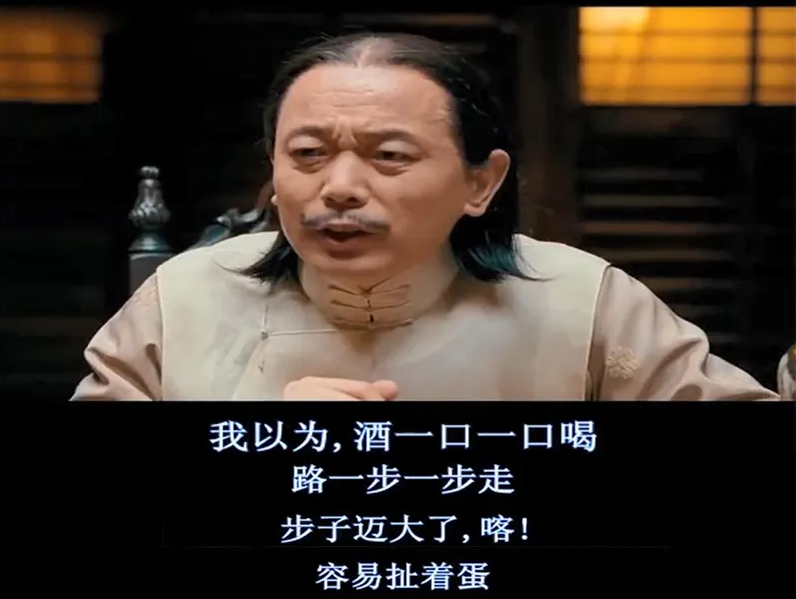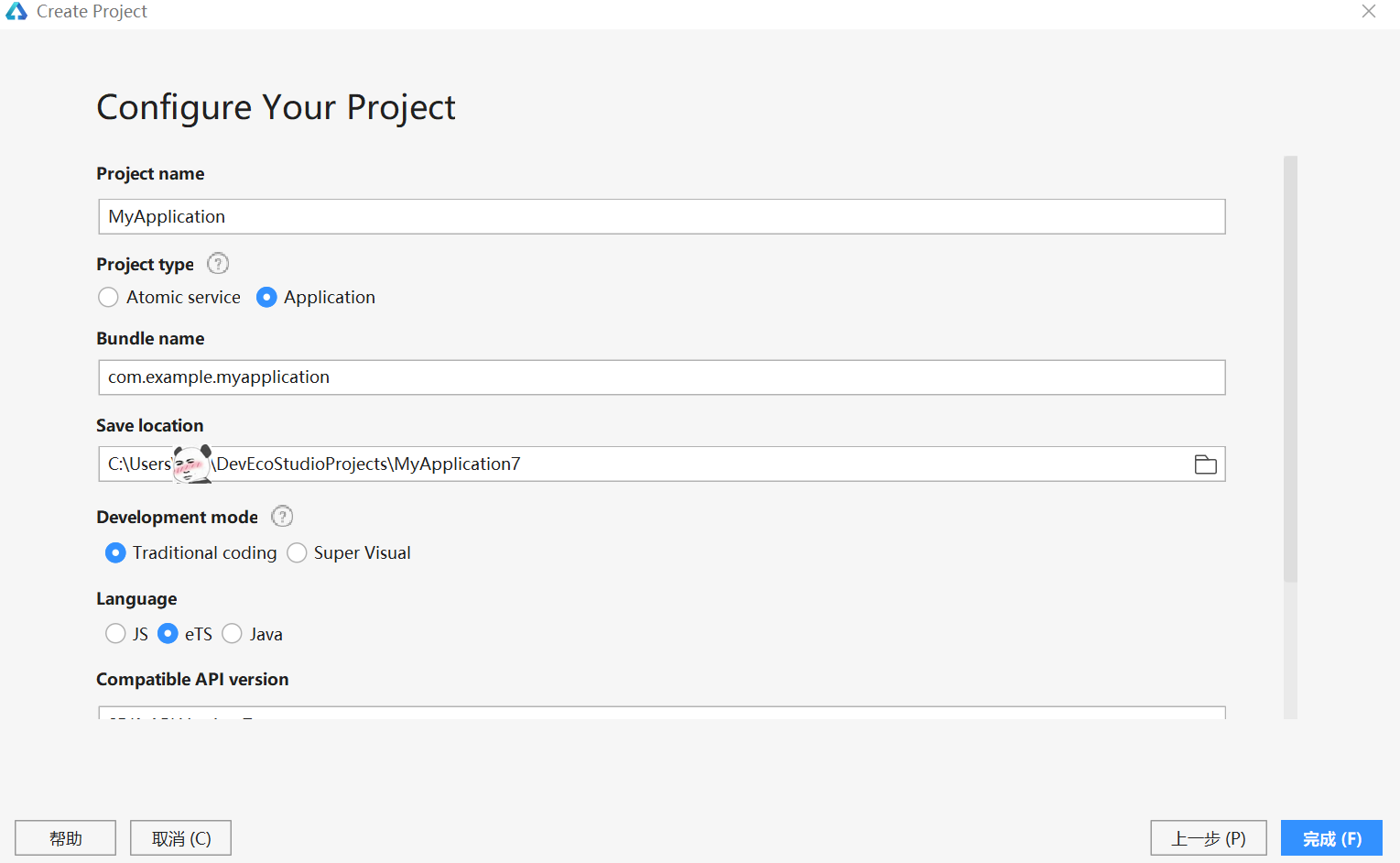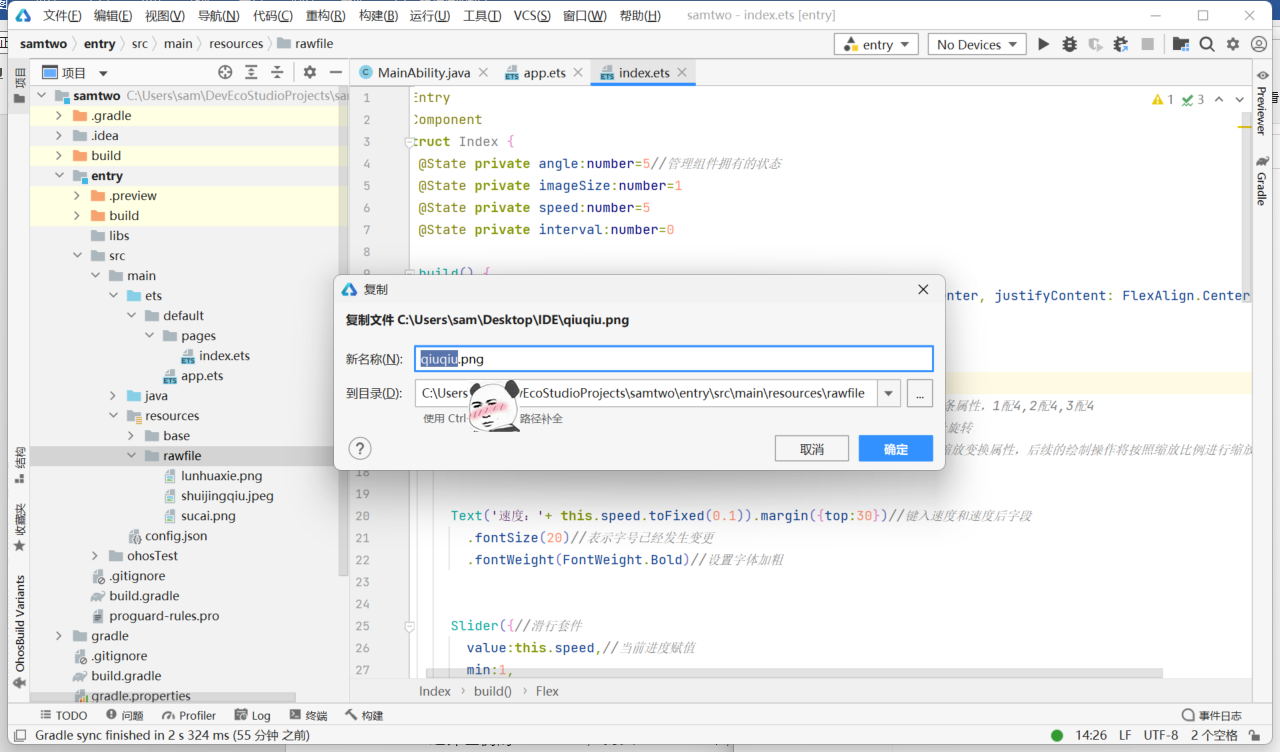# 【HarmonyOS学习笔记】Slider组件实现图形可调旋转 原创

0收藏SDK没有多余选项，目前的IDE只能选择API 7Image(\$rawfile('shuijingqiu.jpeg'))
.objectFit(ImageFit.Contain)
.height(150)
.width(150)
.margin({top:50,bottom:150,right:16})
.rotate({x:0,y:0,z:1,angle:this.angle})
.scale({x:this.imageSize,y:this.imageSize})

@State private angle:number=5

canvs画布的缩放变换属性，以及后续的绘制操作，都需要给定一个初始值

@State private imageSize:number=1

Text('速度：'+ this.speed.toFixed(0.1)).margin({top:30})//键入速度和速度后字段
.fontSize(20)//表示字号已经发生变更
.fontWeight(FontWeight.Bold)//设置字体加粗

@State private speed:number=5

Slider({//滑行套件
value:this.speed,//当前进度赋值
min:1,
max:10,
step:1,
style:SliderStyle.OutSet//滑动模块风格类型，inSet不能在外部拖放
}).showTips(true)//设置滑动时是否显示气泡提示百分比
.blockColor(Color.Blue)//设置滑块颜色

.onChange((value:number)=>{
this.speed = value//Tab页签切换后触发的事件，这里是当切换后将数字赋给speed当前速度值
})

speedChang() {
var that = this

@State private interval:number=0

this.interval = setInterval(function () {
that.angle += that.speed
}, 15)//为避免报错，这里要给定一个时间间隔，否则会报错，我这里设定的是10毫秒

onPageShow(){
this.speedChang()
}

@Entry
@Component
struct Index {
@State private angle:number=5//管理组件拥有的状态
@State private imageSize:number=1
@State private speed:number=5
@State private interval:number=0

build() {
Flex({ direction: FlexDirection.Column, alignItems: ItemAlign.Center, justifyContent: FlexAlign.Center }) {
Image(\$rawfile('shuijingqiu.jpeg'))//引用图片shuijingqiu
.objectFit(ImageFit.Contain)//设置图片的缩放类型
.height(100)//设置高
.width(100)//设置宽度
.margin({top:50,bottom:150,right:16})//设置上下左右边框属性，1-4条属性，1配4,2配4,3配4
.rotate({x:0,y:0,z:1,angle:this.angle})//针对当前坐标轴进行顺时针旋转
.scale({x:this.imageSize,y:this.imageSize})//设置canvas画布的缩放变换属性，后续的绘制操作将按照缩放比例进行缩放

Text('速度：'+ this.speed.toFixed(0.1)).margin({top:30})//键入速度和速度后字段
.fontSize(20)//表示字号已经发生变更
.fontWeight(FontWeight.Bold)//设置字体加粗

Slider({//滑行套件
value:this.speed,//当前进度赋值
min:1,
max:10,
step:1,
style:SliderStyle.OutSet//滑动模块风格类型，inSet不能在外部拖放
}).showTips(true)//设置滑动时是否显示气泡提示百分比
.blockColor(Color.Blue)//设置滑块颜色
.onChange((value:number)=>{
this.speed = value//Tab页签切换后触发的事件，这里是当切换后将数字赋给speed当前速度值
})

Text('缩放大小：' + this.imageSize).margin({top:30})
.fontSize(20)
.fontWeight(FontWeight.Bold)

Slider({
value:this.imageSize,
min:0.5,
max:2.5,
step:0.1,
style:SliderStyle.OutSet
}).showTips(true)
.blockColor(Color.Blue)
.onChange((value:number)=>{
this.imageSize = value
})

}
.width('100%')
.height('100%')
}
speedChang() {
var that = this
this.interval = setInterval(function () {
that.angle += that.speed
}, 15)
}

onPageShow(){
this.speedChang()
}

}

/*小威的技术试水*/
0 error(s),0 warning(s)